# 一、Python用什么表达二维数组

严格意义上说，Python中并没有数组的概念，Python中表达一组数据有多种形式，例如list，tuple，set等数据结构都可以表达一组数，并且这组数也没有C和C++中数组的的同质限制，这些数可以是任何一种数据类型。

以list为例（list又叫列表），要想实现一个所谓的二维数组（有行有列），可以使用嵌套list来实现。例如：要想实现一个如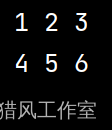``L = [[1, 2, 3], [4, 5, 6]]``

# 二、如何初始化一个二维数组

在很多应用场景下，二维数组的数据是从键盘或者其他什么文件中输入的。这时就迫切需要初始化一个二维数组，等待数据的录入。

## 2.1 利用list自身进行构造

list自身的构造函数形式多种多样。想构建一个二维数组自然并非难事。

例如，想要构建一个m行n列的二维数组。可以这样初始化。

``L = [[] * n] * m``

展示结果如下：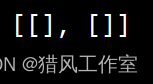如果想要给一个初始化的值，可以写成：

``L = [ * n] * m``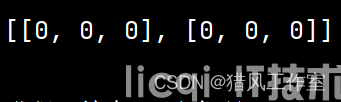## 2.2 利用传统的list的append方法，通过迭代构造

``````m = 2 #2行
n = 3 #3列

l = 0 #行从0开始
mat = [] # 定义一个二维数组mat
while l < m:
r = 0 #列从0开始
line = [] #line存储每一行数据
while r < n:
line.append(0) #装载行数据
r = r + 1
mat.append(line) #按行装载二维数组
l = l + 1
print(mat)``````## 2.3利用numpy包构建二维数组（矩阵）

可以使用numpy包来构建二维数组，例如要构建一个m行n列的初始化为0二维数组，可以编写程序：

``````m = 3
n = 4
mat = np.zeros((m, n))

print(mat)``````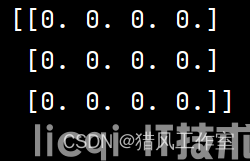类似的：

``````m = 3
n = 4
mat = np.ones((m, n))

print(mat)``````

构建一个m行n列的初始化为0-1随机数的二维数组：

``````m = 3
n = 4
mat = np.random.random((m, n))

print(mat)``````

构建一个m行n列的初始化为1-11步长为2的二维数组：

``````m = 2
n = 3
mat = np.arange(1, 12, 2).reshape(m, n)

print(mat)``````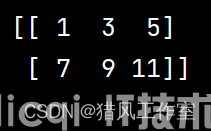``````m = 2
n = 3
mat = np.empty((m, n))

print(mat)``````

但注意，结果并不为空，而是一些极小的浮点数，并没有实际意义。

# 三、操作二维数组

如果你用的是list，那么操作二维数组和其他语言没什么区别。也是通过方括号下标来获取单个数据，也是通过for循环迭代来遍历二维数组。这里不再赘述。2
5
10
20
50